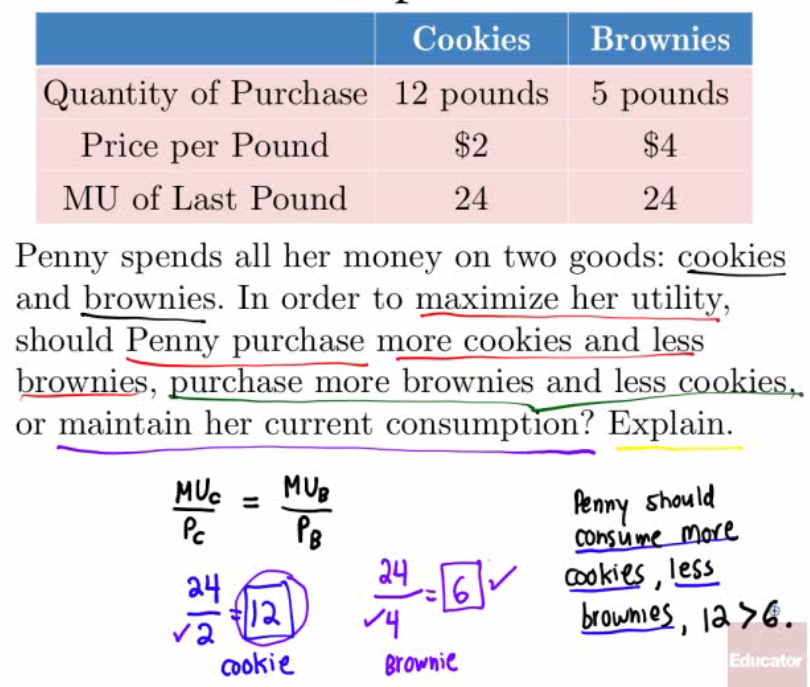# Utility vs. Marginal Utility

• Utility

• arbitrary measure of benefit one receives from an activity (measured in utils)
• Marginal Utility

• change in total utility generated by consuming one additional unit of that good or service

• Air, for example, is necessary for survival but tends to have little value in terms of marginal utility.

• Diamonds, on the other hand, provides lots of marginal utility for many consumer.

# Marginal Utility Per Dollar

• The marginal utility per dollar spent on a good considers budge constrains

• Formula:

•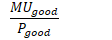• We are constrained by a budget.

• The role of scarcity comes into play when making consumer choices.

• Example:

• We prefer a vacation to Hawaii over a movie, but we must consider the cost of each decision.

• If Hawaii's marginal utility is 500 but costs $500, and a movie's marginal utility is 50 but costs$10, what do we choose?

• Marginal Utility Per Dollar for Hawaii: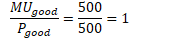• Marginal Utility Per Dollar for movie: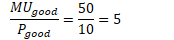• Since 5 > 1, choose movie

# Diminishing Marginal Utility

• As a person increases consumption, there is a decline in the marginal utility from consuming each additional unit of that product.

• You get less "bang for your buck"

• Applies to most, if not all, products at a certain point.

• All You Can Eat restaurant can stay in business because of this principle.

# Optimal Consumption Bundle

• Marginal utility per dollar must be equal for both products

• Formula:

•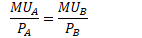• Consumers instinctively follow this rule.

• Within a limited budget, we are required to make choices based on what we value.

• Keep on selecting the item that has the HIGHER marginal utility per dollar.

• Due to diminishing marginal utility, that value begin to fall until equals the marginal utility per dollar for the other item.

• Example 1• Example 2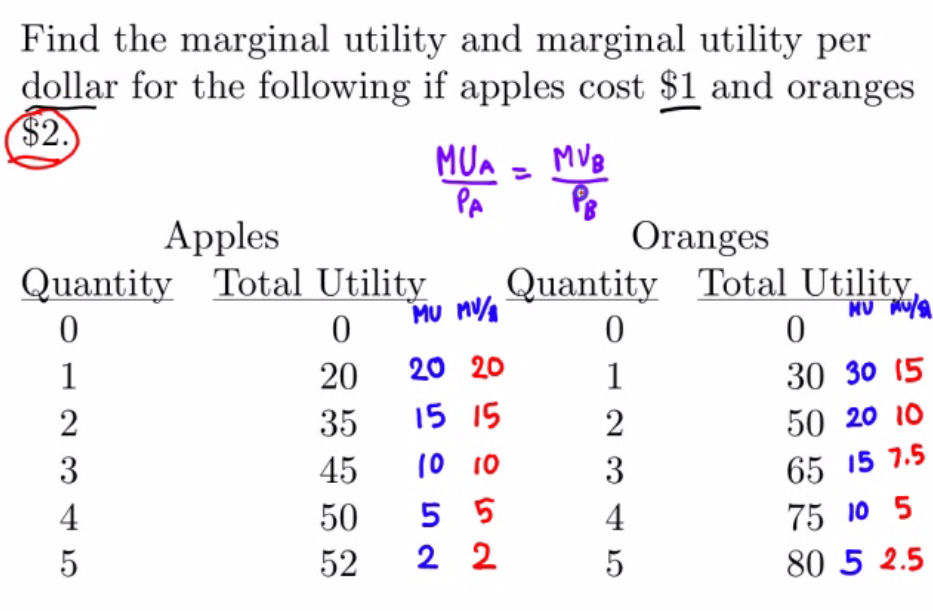• Example 3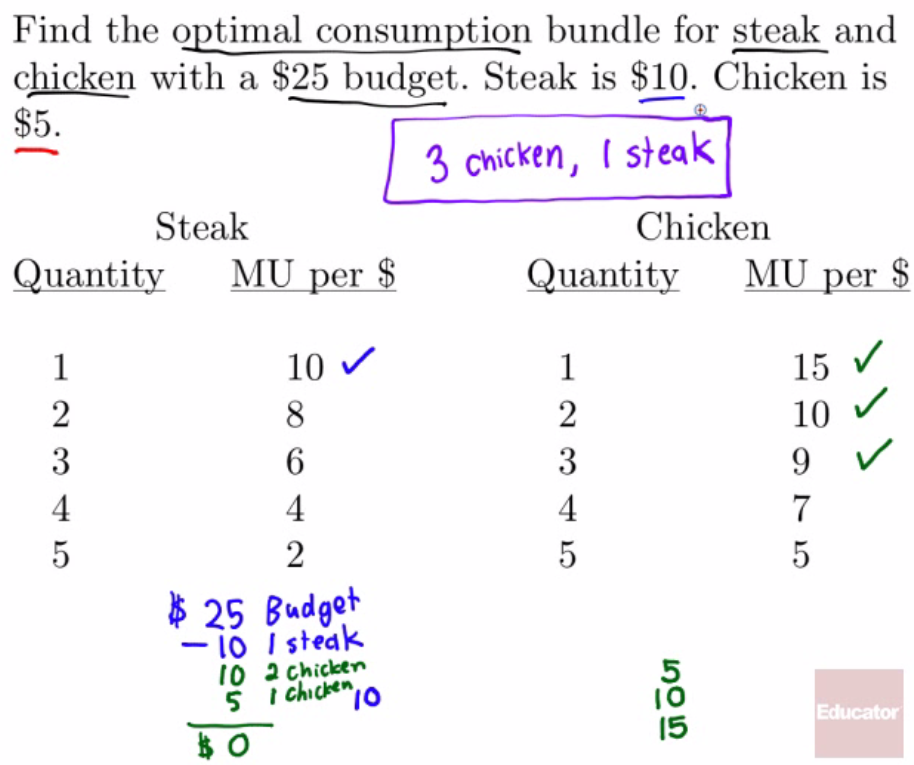• Example 4